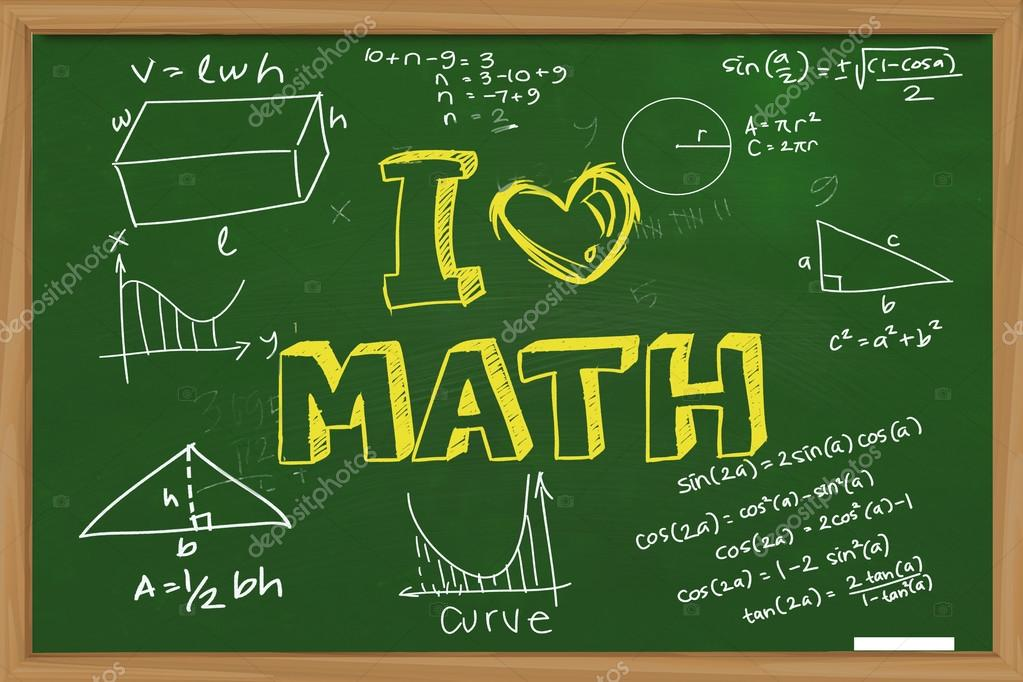#### Math

KG Primary

Mathematics provides a powerful and universal language. Students are expected to use mathematical language appropriately when communicating mathematical ideas, reasoning and findings—both orally and in writing.

We help students to be able to communicate mathematical ideas, reasoning and findings by being able to:

• use appropriate mathematical language (notation, symbols, terminology) in both oral and written explanations
• use different forms of mathematical representation (formulae, diagrams, tables, charts, graphs and models)
• move between different forms of representation.